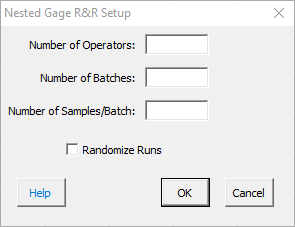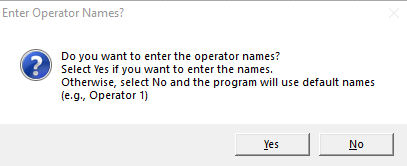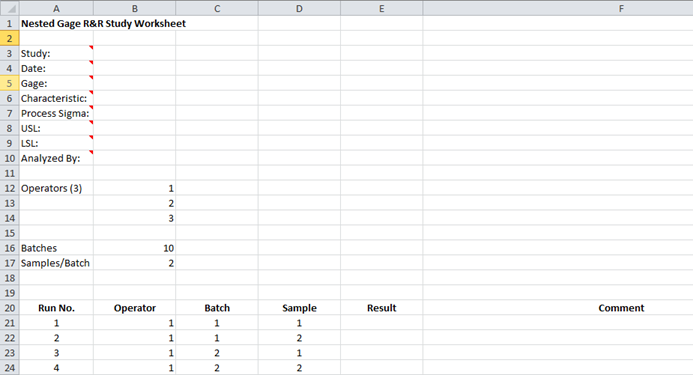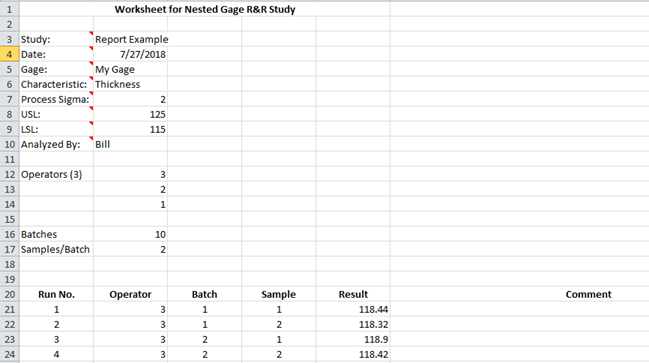# Nested Gage R&R HelpThis help page shows how to setup and run a nested gage R&R study.  The data used in this example can be downloaded at this link.

1. To start the analysis, select “MSA” from the “Analysis” panel on the SPC for Excel ribbon.  Then select “Gage R&R Studies” and “Nested-ANOVA”.

2. The input form below is shown.  Enter the number of operators, batches and samples per batch.  You also have the option to randomize the runs in the study. Select OK.3. The option to enter the operators’ names is given.  If you select No, the software lists each operator as Operator 1, Operator 2, etc.  If you select yes, then an input form is shown for each operator so you can enter the name.4. A nested Gage R&R worksheet is added to the workbook. This is where you enter the information and results.5. Enter the information into the top part of the worksheet.  There is a comment in each cell in rows 3 to 10 in column A that explain the entry. All entries are optional.  Each entry is explained below.

Study: optional,name of study
Date: optional, date Gage R&R performed
Gage: optional, name of gage
Characteristic: optional, characteristic being measured
Process Sigma: optional, value used for the total variance instead of the calculated total variance of the Gage R&R results
USL: optional, used to determine % of tolerance explained by study variation
LSL: optional, used to determine % of tolerance explained by study variation
Analyzed By: optional, name of person doing the analysis

6. Enter the results for each combination of operator, batch and sample.

7. Part of a completed nested gage R&R worksheet is shown below.8. The results are now ready to be analyzed using the ANOVA method. To analyze the results, select “MSA” from the “Analysis” panel on the SPC for Excel ribbon.

9. The following options are displayed.The options are explained below.

• Two additional charts can be created
• Run chart by part
• Run chart by operator
• Sigma Multiplier: the value that standard deviation is multiplied by. The default value is 6.  This captures 99.73% of the data.  5.15 is sometimes used. This value captures 99% of the data.
• Detailed or Summary Report: both are displayed on single worksheet. The detailed report contains all the charts, calculations and explanation of results.  The summary report contains the charts.
• Data Table: the option to include a data table in the report.

Select OK to generate the report in the workbook.

10. A pdf file containing the detailed report with a description of the output is given at this link.  The output includes:

• ANOVA table
• % Contribution based on variance
• % Based on standard deviation
• Number of distinct categories
• Variance components chart
• Operator-Batch Control Charts
• Control chart calculations
• X chart analysis
• R chart analysis
• Run chart by operator (if option selected)
• Run chart by batch(operator) – (if option selected)
• Data table